#Interactive Real Analysis

Next | Previous | Glossary | Map

## 3.5. Special Sequences

### Definition 3.5.6: Euler Sequence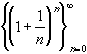Euler's Sequence: Converges to e ~ 2.71828182845904523536028747135... (Euler's number). This sequence serves to define e.

Euler's sequence

### Proof:

We will show that the sequence is monotone increasing and bounded above. If that was true, then it must converge. Its limit, by definition, will be called e for Euler's number.

Euler's number e is irrational (in fact transcendental), and an approximation of e to 30 decimals is e ~ 2.71828182845904523536028747135.

First, we can use the binomial theorem to expand the expression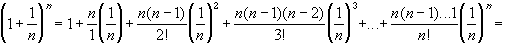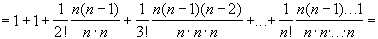Similarly, we can replace n by n+1 in this expression to obtainThe first expression has (n+1) terms, the second expression has (n+2) terms. Each of the first (n+1) terms of the second expression is greater than or equal to each of the (n+1) terms of the first expression, because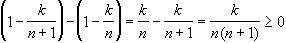But then the sequence is monotone increasing, because we have shown that-0
Next, we need to show that the sequence is bounded. Again, consider the expansion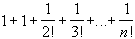1 +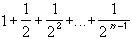Now we need to estimate the expressionto finish the proof.

If we define Sn =, then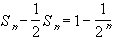so that, finally,for all n.
But then, putting everything together, we have shown that1 +1 + Sn3
for all n. Hence, Euler's sequence is bounded by 3 for all n.

Therefore, since the sequence is monotone increasing and bounded, it must converge. We already know that the limit is less than or equal to 3. In fact, the limit is approximately equal to 2.71828182845904523536028747135Next | Previous | Glossary | Map